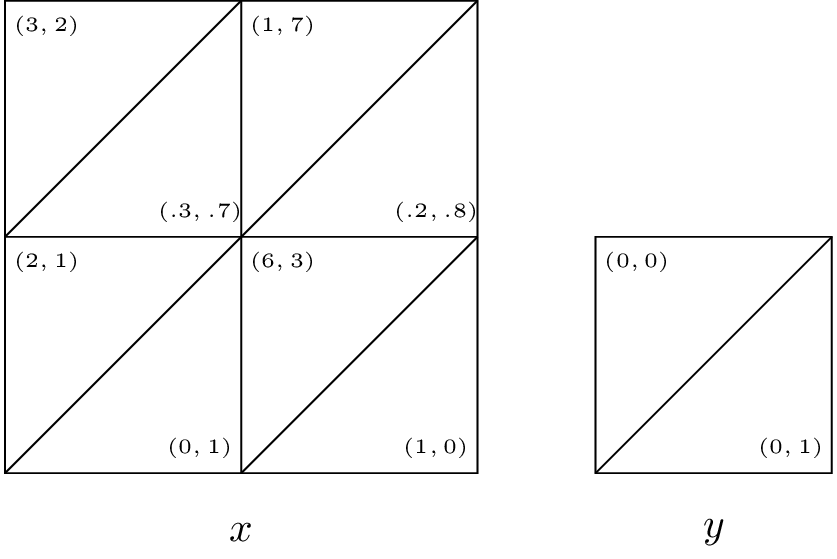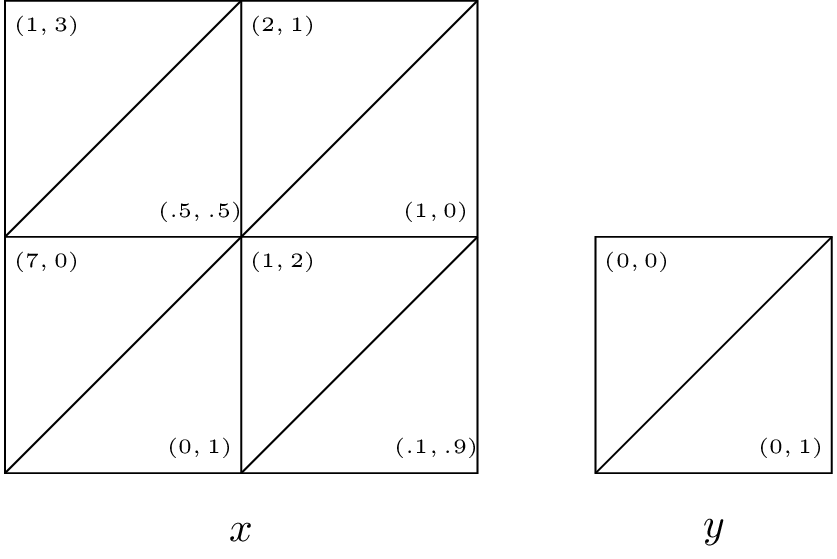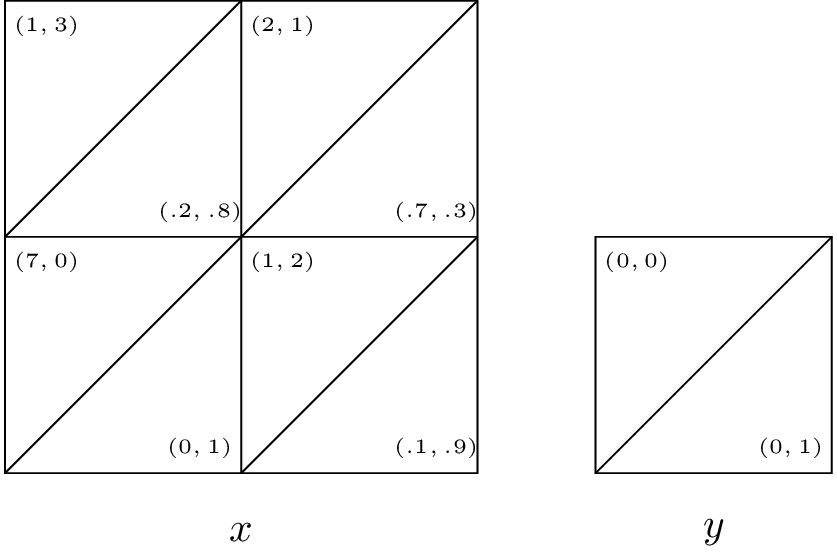1. Consider the pairwise contest games with the following associated two player games:

Identify all evolutionary stable strategies.

2. Consider the following game:

In a mathematics department, researchers can choose to use one of two systems for typesetting their research papers: LaTeX or Word. We will refer to these two strategies as $$L$$ and $$W$$ respectively. A user of $$W$$ receives a basic utility of 1 and as $$L$$ is more widely used by mathematicians out of the department and is in general considered to be a better system a user of $$L$$ gets a basic utility of $$\alpha > 1$$. Members of the mathematics department often collaborate and as such it is beneficial for the researchers to use the same typesetting system. If we let $$\mu$$ represent the proportion of users of $$L$$ we let:

$u(L,\chi)=\alpha+2\mu$ $u(W,\chi)=1+2(1-\mu)$

What are the evolutionary stable strategies?

3. Consider the following two normal form games:

Assume both players play either game $$A$$ or game $$B$$ with probability $$1/2$$, neither player knows which game is played. Obtain the Nash equilibrium for this game.

4. Repeat the analysis of the principal agent game assuming that $$p$$ is the probability of the project being successful in case of a high level of effort by the employee.

1. What are the expected utilities to the employer and the employee?
2. Obtain a condition for which the employer should offer a bonus.
5. Obtain the Markov Nash equilibrium (in pure strategies if it exists) for the following games assuming $$\delta=1/4$$.Solution available